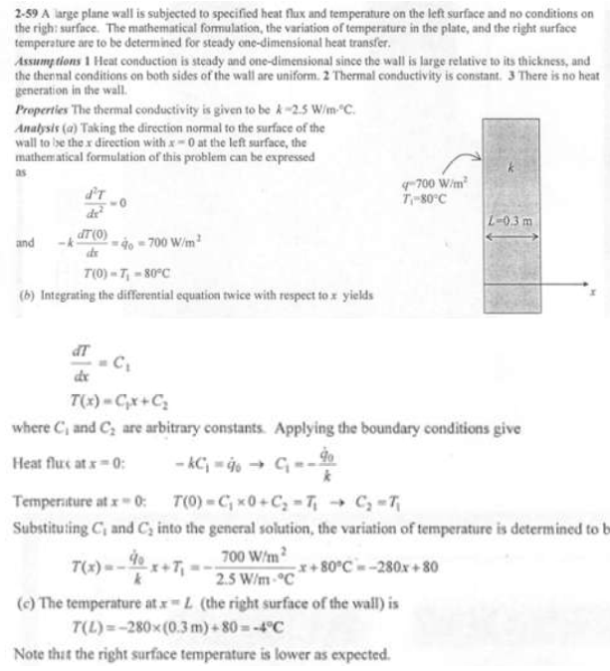heat and mass transfer problems engineering equations heat and mass transfer school homework engineering heat and mass transfer formulas heat and mass transfer solutions to heat and mass transfer problems full solution engineering problem solution heat and mass transfer math problems engineering equations heat and mass transfer school homework engineering solutions to heat and mass transfer formulas heat problems mass problem solutions to transfer problems full solution heat and mass transfer
heat and mass transfer problems engineering equations heat and mass transfer school homework engineering heat and mass transfer formulas heat and mass transfer solutions to heat and mass transfer problems full solution engineering problem solution heat and mass transfer math problems engineering equations heat and mass transfer school homework engineering solutions to heat and mass transfer formulas heat problems mass problem solutions to transfer problems full solution heat and mass transfer
Highalphabet Home Page heat and mass transfer problem solutions Heat and Mass Transfer Page
Consider a large plane wall of thickness L=0.3 m, thermal conductivity k=2.5 W/mK, and surface area A=12 m^2. The left side of the wall at x=0 is subjected to a net heat flux of 700 W/m^2 while the temperature at that surface is measured to be 80 C. Assuming constant thermal conductivity and no heat generation in the wall, (a) express the differential equation and the boundary conditions for steady one-dimensional heat conduction through the wall, (b) obtain a relation for the variation of temperature in the wall by solving the differential equation, and (c) evaluate the temperature of the right surface of the wall at x=L.Consider a large plane wall of thickness L=0.3 m, thermal conductivity k=2.5 W/mK, and surface area A=12 m^2. The left side of the wall at x=0 is subjected to a net heat flux of 700 W/m^2 while the temperature at that surface is measured to be 80 C. Assuming constant thermal conductivity and no heat generation in the wall, (a) express the differential equation and the boundary conditions for steady one-dimensional heat conduction through the wall, (b) obtain a relation for the variation of temperature in the wall by solving the differential equation, and (c) evaluate the temperature of the right surface of the wall at x=L.